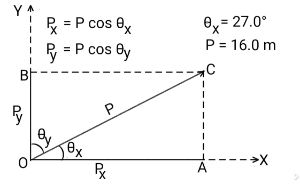# Calculate the x-component and the y-component (in m) of the vector with magnitude 16.0 m and...

## Question:

Calculate the x-component and the y-component (in m) of the vector with magnitude 16.0 m and direction 27.0{eq}^{\circ} {/eq}.

## Mutually Perpendicular Vector Components:

When a vector is broken into two or more mutually perpendicular vector components, the vector components can be added vectorially to get the original vector.

If a vector component of a vector makes an angle {eq}\theta {/eq} with the original vector {eq}\vec P {/eq}, the magnitude of this component vector will be given by:

{eq}\displaystyle{p = |\vec P| \cos \theta} {/eq}.Given

• The magnitude of the vector: {eq}P = 16.0/\ \rm m {/eq}.
• The angle the vector makes with the +ve x-direction: {eq}\theta_x = 27.0^\circ {/eq}.

Therefore, the angle the vector makes with the +ve y-direction will be given by:

{eq}\displaystyle{\begin{align*} \theta_y &= 90.0^\circ - \theta_x\\ \theta_y &= 90.0^\circ - 27.0^\circ\\ \theta_y &= 63.0^\circ\\ \end{align*}} {/eq}

The magnitude of the x component of the original vector will be given by:

{eq}\displaystyle{\begin{align*} P_x &= P \cos \theta_x\\ P_x &= (16.0 \ \rm m)\times \cos 27.0^\circ\\ P_x &\approx \boxed{{14.3 \ \rm m}}\\ \end{align*}} {/eq}

The magnitude of the y component of the original vector will be given by:

{eq}\displaystyle{\begin{align*} P_y &= P \cos \theta_y\\ P_y &= (16.0 \ \rm m)\times \cos 63.0^\circ\\ P_y &\approx \boxed{{7.26 \ \rm m}}\\ \end{align*}} {/eq}